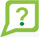We matched that to:

## How do I calculate the margin required for a long stock purchase or short sell?

To calculate the margin required for a long stock purchase, multiply the number of shares by the price by the margin rate. The margin requirement for a short sale is the margin requirement plus 100% of the value of the security.

Margin Requirement = shares x price x margin rate percentage

Examples:

### Short Stock

Purchase 1,000 shares of a stock at \$50 with margin rate of 30%. The margin requirement would be:

1,000 shares x \$50 x 30% margin rate = \$15,000

This is the minimum required amount + margin that must be in the account before a buy order can be entered.

Since 30% is the margin rate, TD Direct Investing is lending the account holder 70% of the trade value.

Maximum loan value is 1,000 shares x \$50 x 70% = \$35,000
Short sell 500 shares of a 50% marginable stock priced at \$10.00. The margin requirement is 150%. Note: 100% of the margin requirement is generated from the sale of the security. Therefore, the additional initial margin requirement is 50%, the same amount required in order to accept the trade if you were purchasing the stock.

500 shares x \$10.00 x 50% = \$2,500

The total margin requirement to hold the position, including the 100% of the proceeds from the short sell, is calculated as follows:

500 shares x 10.00 x 150% = \$7,500
(Note: TD Direct Investing does not pay interest on the cash amount that a client receives as a result of a short sale.)

You may also try:

### Top 10 FAQs